# IMPACT Live!

View Only

## IMPACT Plus #2 - Teaching students to make sense of mathematical symbolism

####By Claire Wladis posted 05-10-2021 01:35:21

We know that students struggle with abstract mathematical symbolism at all levels, starting in elementary school, and continuing through various levels in college. Students often work with symbols as though the goal were to move them around on the page in order to satisfy arbitrary rules. These are rules they have adopted from their prior instructional experiences without really understanding how, why, or when they work. Students may even believe that it is not possible to understand the rules that they use—they may view the procedures like allowed chess moves, something that experts know and which must be memorized, but that cannot be derived or explained on one’s own.

In our research, we have been interested in trying to understand what core knowledge is necessary for students to work with abstract mathematical symbolism, thinking in particular about ideas that are relevant across many levels of mathematics instruction, but focusing primarily on algebra since it is a core area where students first have to use more complex abstract symbolism. In order to better understand this, we have observed student work in classrooms, interviewed hundreds of students at all levels of college mathematics, from elementary algebra through linear algebra; we have also administered open-ended questions to over 800 students, and multiple-choice questions to thousands of students. We have tried to pay particular attention to both how students tend to conceptualize core ideas of mathematics, as well as how this might differ from how most mathematicians think about these concepts. In this we focus not on mathematical modeling, which has already been the focus of much research, but rather on the less examined area of how students work with abstract symbolic mathematics, used in computational tasks such as simplifying expressions and solving equations.

Abstract symbolic mathematics has a meaning all its own, different from, but equally important in comparison to other skills like modeling. If we want to understand how to best support students in using abstract mathematical symbols, one place to start is to ask ourselves how we can connect the ways that mathematicians use symbols to the way students use symbols, in order to give students equitable access to formal mathematics.

We have identified three core ideas as critical to using abstract mathematical symbolism productively:

1) Syntactic structure: How do students “read” the meaning of the syntax of expressions and equations (and other objects)?

2) Using properties: How do students justify their transformation steps (e.g., when simplifying)?

3) Equivalence: Do students have a well-defined notion of equivalence appropriate to the specific context, and do they connect it to the justification of their computational work?

For the purposes of this blog post, we will explore how just this third core idea might impact student understanding of simplification. We do this through the lens of one student’s work on the following algebra problem: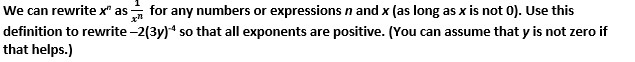This question is typical of standard questions given to college students in Elementary Algebra (the first level of developmental algebra, similar in content to Algebra I in high school). Students often run into trouble with this and similar questions, largely because they try to perform mathematically-invalid symbolic manipulations.

## Do students justify transformation with equivalence? Do they use substitution equivalence?

Equivalence is one of the core ideas across all domains of mathematics. We could argue that many every single mathematical task involving symbolic manipulation is fundamentally focused on either generating equivalent objects, or on determining whether two objects are equivalent. But the definition of equivalence changes, depending on the mathematical context, even within a single subject or domain. Yet we rarely define equivalence explicitly during instruction (and if we do, we rarely return to the definition explicitly, or explicitly note its central role as we teach students to simplify or solve problems). This can be an issue if students do not connect the notion of equivalence to the computational work that they do, understanding that every transformation is only “allowed” by definition because it preserves equivalence.

When simplifying expressions, the underlying notion of equivalence that has to be preserved by all transformations is insertion equivalence (Prediger & Zwetzschler, 2013), or in other words: two expressions are equivalent if and only if they both have all the same values, no matter which numbers we substitute in for each of the variables (obviously assuming that the same values get substituted in for the same variables in each expression). However, it is not clear that students necessarily connect any of their computational work to the notion that all their computations should preserve the insertion equivalence of the expressions that they are transforming.

We have one sample student’s work that we want to present as an illustration to think about how they might be conceptualizing equivalence (and then later, substitution equivalence, more specifically).

### Does this student view equivalence as a computational process or a relationship between objects?

Let’s first consider this student’s use of the equals sign in their work: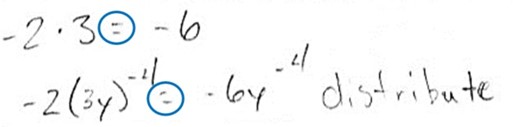This student used the equals sign twice: in the first line, to indicate that –6 is the product of –2 and 3, and then again in the second line, where they have used the word “distribute”. This use of ‘distribute’ seems to describe an incorrect simplification that is not an example of the distributive property, but which resembles it if we think of distributing as “taking what is outside the parentheses and multiplying it by what is inside”. In the first line, it is possible that the student is thinking of the equals sign as a symbol that indicates the result of a computation, or ‘operational conception’, rather than as a symbol that represents a relationship, or ‘relational conception’, which has been observed frequently in research with elementary and middle school students, (Knuth et al., 2006) as well as in our research with college students. We can’t be sure simply from the work written here whether this student is viewing (or is able to view) the equals sign as indicating a relationship or not, but we note that the student’s work throughout this set of questions tended to imply that they are likely primarily focused on the results of computation rather than relationships.  For example, consider their work on the second line. They have written the word “distribute” to the right, suggesting that they see what is on the right of the equals sign as the result of a “distribute” computation performed on the left of the equals sign. This is reinforced by its placement directly under the arithmetic computation on the first line, and its parallel nature to what is in the first line, where the multiplication in the first line could be seen as justifying the multiplication in the second line, where the focus is in justifying the product of –2 and 3y. The student does not appear to be focused at all on reasoning about the structure of the expression –2(3y)–4 in this line.

### Does this student see the result of each step in their work as equivalent to the one before?

We don’t have a definitive answer to this question from the student’s work here, but it can be informative to look at how this student handled the equals signs in each line. We notice, for example, that there is no explicit link in their work between one step and the next. They have written two equations in the first two lines, but have not indicated the relationship between those equations explicitly. For example, the expressions written in the first line are not equivalent to those in the second line. In particular, if we look at the third line of their work, this student has left off any kind of equals or equivalence sign: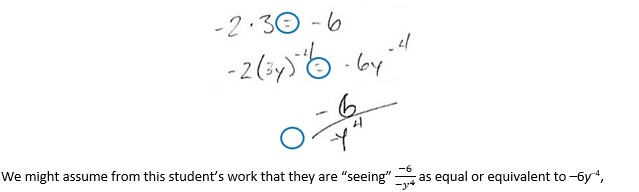but it is not actually clear that they think about equivalence or equality at all when they are performing this simplification. We have found that it is quite common for students to leave out key equals signs such as this in their work when they are simplifying expressions, and that this tends to correlate with students viewing ‘simplifying’ as a process of using procedures to change one expression into another, and where they do not connect the process of simplifying to the notion of equivalence. We cannot rule out the fact that this student might have left off the equals sign accidentally, so we cannot be certain how this particular student might think about equivalence. We simply note that these patterns were common among the students we studied, and that the lack of equals signs can sometimes be a clue that students do not connect their computational work to underlying equivalence relationships.

We don’t have detailed information about how this student is defining equivalence in their work, but we digress for a brief moment just to outline briefly some of the ways in which a student’s definition of equivalence might impact their thinking.

#### A brief digression: To what extent do students have explicit and well-defined notions of equivalence?

We use the word equivalent a lot in standard algebra curricula in both K-12 and college contexts, yet we have rarely seen this word explicitly defined in textbooks, curricula, or by instructors that we have observed. Equivalence is a cross-cutting idea in mathematics, but it is not used the same way in every domain, even within a single topic it might take on multiple meanings.

For example, in algebra, here are some common definitions of equivalence that are often confused by students:

Equivalent expressions: Usually this means that two expressions are insertionally equivalent, or that they both produce the same answer every time we substitute in the same numbers for the same variables in each expression.

Equivalent equations: Usually this means that two equations have the same solution set. Students will often confuse this definition with equivalent expressions, for example, saying that 2x + y = 3 and xy = 3 are equivalent because “they equal the same number”.

Equivalent forms: Two equations may have the “same form”, for example, if they both are linear, or have the form y = mx + b. Having the “same form” is also a type of equivalence, even though we often don’t use that word in the curriculum. However, students often notice that both “same form” and “equivalence” describe a kind of structural sameness, even when their thinking is not completely robust, and therefore they will often confuse the notion of “same form” with equivalent equations, and say, for example, that the equations y = 2x + 1 and y = 3x + 4 are equivalent because they have the “same form”.

It is not clear which, if any, of these definitions of equivalence are at play in the student’s mind while they were doing the work that we have been discussing here. However, if they had a well-defined definition of equivalent expressions in mind (e.g., as described above), and they connected that idea to their computation, they might notice that some of their work does not preserve equivalence. For example, they could check whether –2(3y)–4 and –6y–4 really produce the same outcomes for the same values of y.

One particularly important type of equivalence in our framework is substitution equivalence. We could think of ALL transformation in algebra (and other domains that use mathematical symbolism, even arithmetic) as a process of substitution equivalence:

No matter which definition of equivalence that we are using, if we replace a subexpression of an expression with another equivalent subexpression, and then the resulting expression will be equivalent to the original one. This process can actually be easy for students to visualize if they are able to identify the correct subexpressions (or if we identify them in advance as a part of a scaffolded question). Even small children can understand the idea that switching one part of something out for another equivalent part preserves equivalence of the whole. The tricky part for algebra students is to check the following three features of this substitution:

1) What is being substituted out must be a subexpression, or a unified whole; and

2) What is being substituted in must be written in such a way that it will also be a subexpression (or unified whole) within the new expression; and

3) What is being substituted in must be equivalent to what is being substituted out.

### Does this student “see” substitution equivalence?

With this in mind, we now ask ourselves: Is this student using substitution equivalence in their work? It is not possible to tell for sure, but there are a few different ways of thinking about their work. Let’s look again at the first two lines: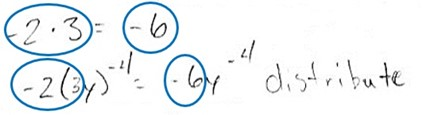We could theoretically think of this as a kind of substitution equivalence: it is possible that the student thought of this as substitution equivalence if they thought of the circled parts in the top row as showing that ‘–2(3’ could be substituted out and –6 could be substituted in in the second row. If they were thinking this way, they were not identifying the correct subexpressions, because ‘–2(3’ is not a subexpression (it is missing half of a set of parentheses and is therefore incomplete), but it is possible that the student does not know that. Another possible explanation, however, is that this student sees the first line as a general formula or process for how to calculate the “distributive property” in the second row, and that they see the first row as a kind of parallel set of computations to the second row, and not as a justification for substitution. We see the second explanation as a more plausible explanation in this case, for several reasons. For example, the computational description of this student’s work (“distribute”) suggests to us that the student is computing something in the second line, and not conceptualizing their work as a kind of substitution. But secondly, on another set of questions on this same test, when specifically asked whether a number of different simplification computations were a kind of substitution, this particular student often answered “no”. So, we suspect that this student is not thinking of their work here as a kind of substitution equivalence. This leads us to ask a few questions:

• If this student were to re-conceptualize their simplification work as a process of substitution equivalence, would they make these kinds of mistakes?
• What kinds of knowledge are necessary for a student to think about their simplification work as a process of substitution equivalence (instead of as an arbitrary collection of procedures)?

We now explore one way that someone might use the ideas of syntactic structure, properties, and substitution equivalence to solve this simplification problem. This is just one possible way that someone might approach this problem structurally, but we present it as an example, as a contrast to the student’s work above.

## Simplifying expressions using a structural approach

To begin with, we might want to rephrase how we even ask the question, to focus on the core notion of equivalence which underlies the work:

Give another expression that is equivalent to 2(3y)–4, but that does not have a negative exponent.

(We note that before we can give students questions phrased this way, we need to have discussed with them more explicitly what the word “equivalence” means in mathematics more broadly, or in the specific context of this particular problem specifically.)

How might we proceed to solve this problem structurally?

##### We first have to “see” 2(3y) –4 as having a structure that is broken into subexpressions: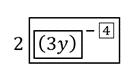We note that we are defining subexpressions here as any substring that can be treated as a unified whole (e.g., by inserting parentheses around it) without changing the syntactic meaning of the expression (i.e., we will still do all the same computations in the same order).

Once we recognize that 2(3y) –4 has this structure, then we can reframe the problem as a search for a subexpression that does not have a negative exponent and that is equivalent to the subexpression

(3y) –4. If we can find an expression that is equivalent to (3y) –4, then we can replace (3y) –4 in the larger expression 2(3y) –4 with that expression: the result will be equivalent to the original expression 2(3y) –4.

##### How do we generate a subexpression that is equivalent to (3y) –4?

We need to use the property: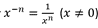Then replacing the subexpression (3y) –4 with the equivalent subexpression generated by this property will preserve the equivalence of the larger expression 2(3y) –4.  A property is a statement which tells us that we can take whatever is on one side of the equation, and replace it with whatever is on the other side of the equation, because the two sides of the equation are by definition equivalent to one another.  (We note that we use the word property broadly, to describe any statement of equivalence which uses more generalized structural forms, and not just to describe things we have traditionally called properties such as the associative or distributive properties.  This is why we call the definition of negative exponents a property.)

##### How do we use this property?

We have to map each subexpression in (3y) –4 to a variable in the form xn (because this is one side of the equation for the property that we are using). This has to be done in a way that is one-to-one (i.e., no symbol in either (3y) –4 or xn can be left out), and we have to be sure that every symbol is mapped to a symbol with the same syntactic meaning. This is a kind of matching game, not dissimilar to tasks we ask young children to perform, just using more abstract mathematical symbols. We find that once the correct subexpressions are identified, students are able to use this “matching” idea to use properties more structurally and productively.

One way to have students do this can be quite simple and visual, for example, using circles, arrows, and different colors to highlight the equivalent structures. Here is one way that we have experimented with this kind of work with students: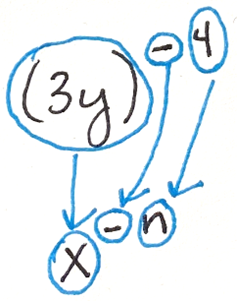Using the circles we can see that:

1) Nothing is left out (everything in both expressions is inside a circle); and

2) Each “thing” in a circle is mapped to something that is equivalent to it. For example, the negative sign is mapped to a negative sign, and not to something else with a different meaning, like a plus sign, or a multiplication sign.

This mapping we have drawn will only work if x = (3y) and n = 4.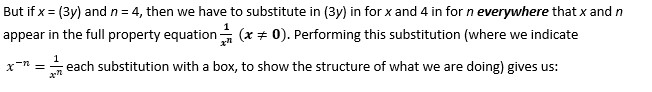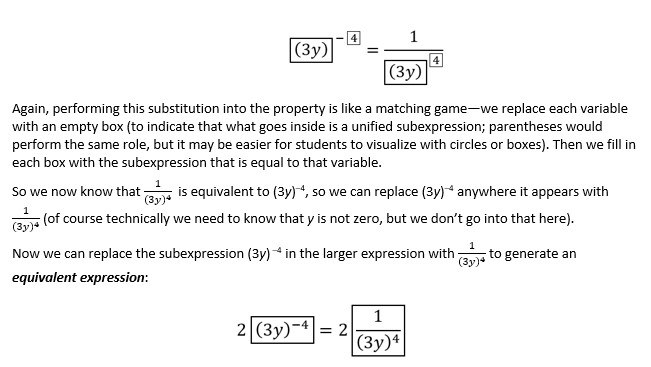While it may not be necessary for students to work out this problem in exactly the structural way that we have discussed above in order to get a correct answer, we hypothesize that an explicit attention to structure and equivalence is necessary if students are actually to be able to learn generalizable computational skills that transfer from one context to another. Exactly how this should be done in developmentally appropriate ways at different points in the curricula is still being researched, and we cannot give anyone definitive recommendations at this time about how we should change the curriculum. However, we believe that discussing these concepts may give instructors new ideas of their own about how they might want to approach their own instruction on simplification and solving, and this might lead to improved instruction in the meantime as we research these ideas further. We also welcome further discussions with instructors which might better inform our future research, so that we can ask the most productive research questions as we continue with this work.

We wind up our discussion here with some other questions that we would like to consider.

## Questions:

• Is it possible, or likely, that students will make many of the most common simplification mistakes (e.g., invalid “canceling”; inappropriate use of “distributing”; “losing” parts of expressions or equations during computation), if they are truly thinking structurally (i.e., trying to map the structures of the expressions they are simplifying to properties in a one-to-one fashion, while thinking directly about equivalence)?
• To what extent might this kind of thinking be helpful to students as they simplify expressions or solve equations?
• To what extent might this kind of thinking be helpful to students in classes beyond algebra, for example as they work with trig identities, perform function composition, do u-substitution, or use the chain rule in calculus?
• Can you identify other ideas that are critical to thinking structurally that are often conveyed implicitly, instead of explained to students explicitly? And if so, can you think of ways to address these important concepts with students more explicitly during instruction?

Bibliography

Prediger, S., & Zwetzschler, L. (2013). Topic-specific design research with a focus on learning processes: The case of understanding algebraic equivalence in grade 8. Educational design research: Illustrative cases, 407-424.

Knuth, E. J., Stephens, A. C., McNeil, N. M., & Alibali, M. W. (2006). Does understanding the equal sign matter? Evidence from solving equations. Journal for research in Mathematics Education37(4), 297-312

#RG-ResearchGrants
#Ownership